### Home > MC1 > Chapter 3 > Lesson 3.4.3 > Problem3-120

3-120.
1. . What number is represented by each of the letters on the number lines below? Homework Help ✎

1.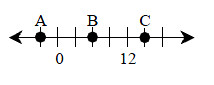2.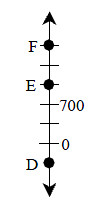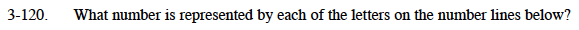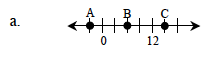Count the number of marks from one given number to the next.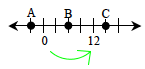There are four marks from 0 to 12. How much does each mark represent?

If four sections represent a total of 12, then each unit must represent 3.

Use the knowledge that the marks are made every 3 integers to determine what A, B, and C represent.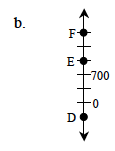D represents −350. Why does that make sense? Now use that to figure out the values of the other points.